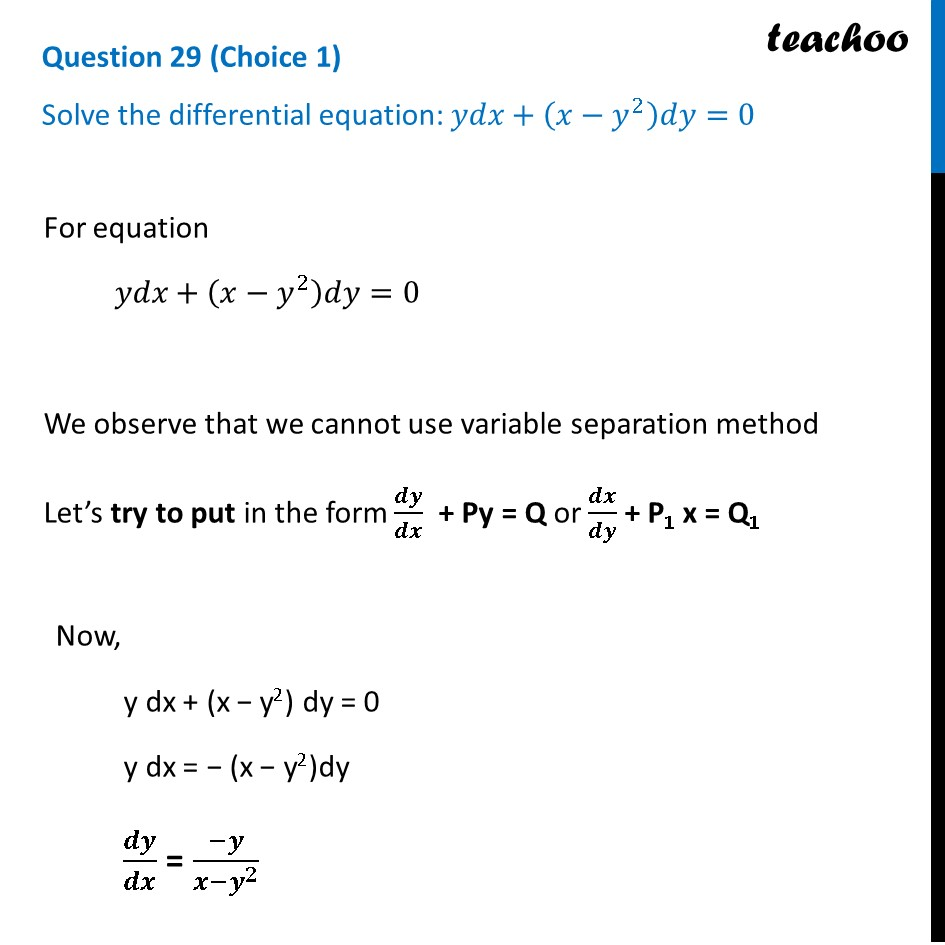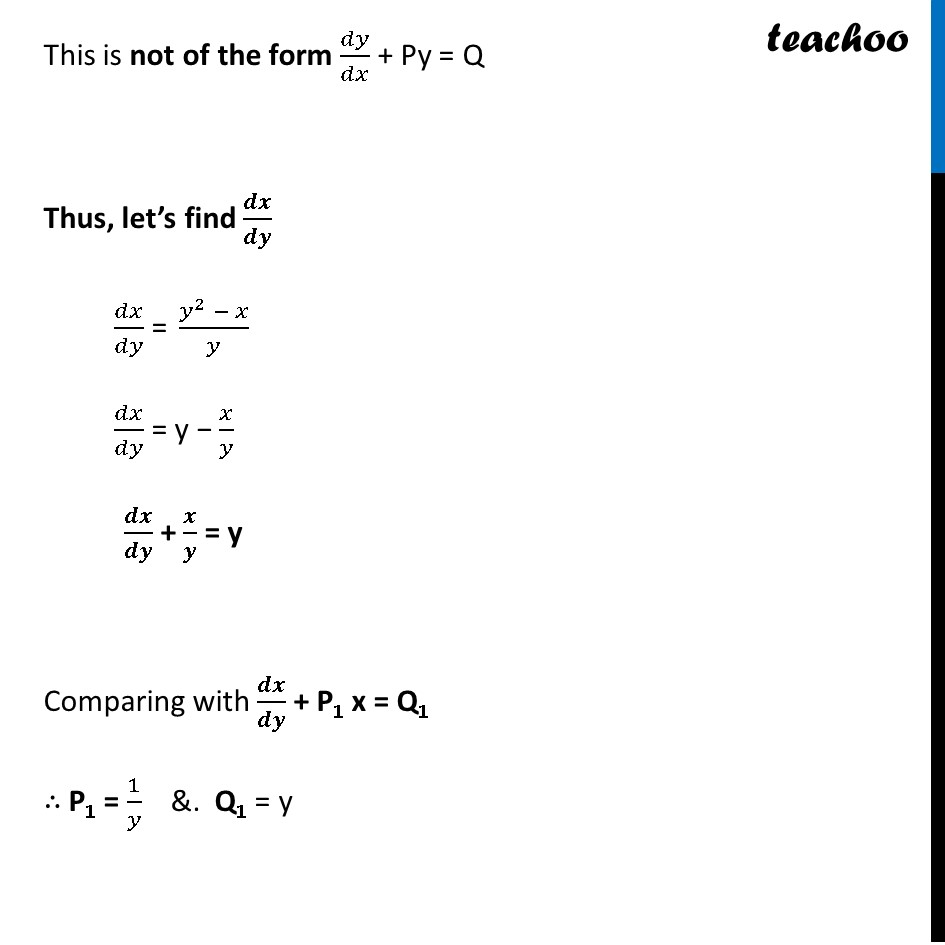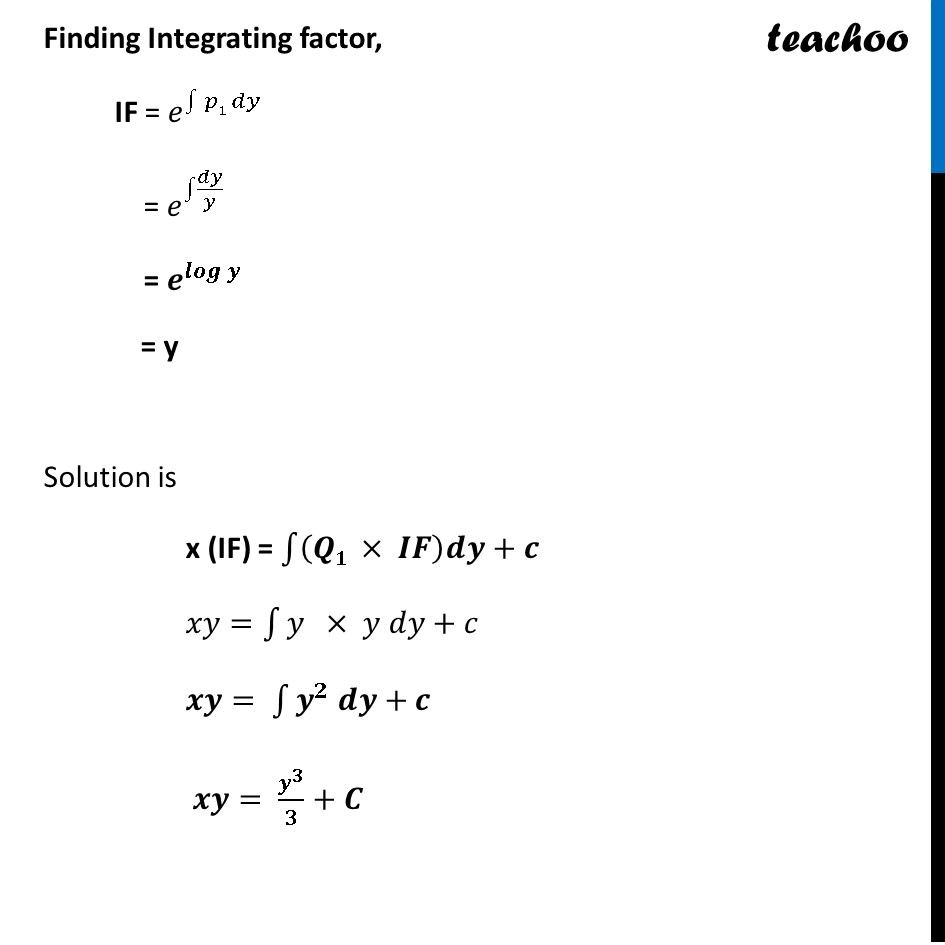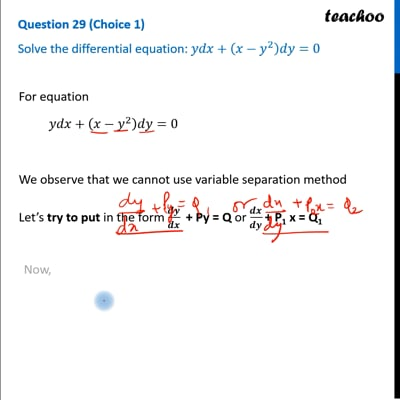CBSE Class 12 Sample Paper for 2023 Boards

Class 12
Solutions of Sample Papers and Past Year Papers - for Class 12 Boards

## Solve the differential equation: ydx+(x-y 2 )dy=0This video is only available for Teachoo black users

Learn in your speed, with individual attention - Teachoo Maths 1-on-1 Class

### Transcript

Question 29 (Choice 1) Solve the differential equation: 𝑦𝑑𝑥+(𝑥−𝑦^2 )𝑑𝑦=0 For equation 𝑦𝑑𝑥+(𝑥−𝑦^2 )𝑑𝑦=0 We observe that we cannot use variable separation method Let’s try to put in the form 𝒅𝒚/𝒅𝒙 + Py = Q or 𝒅𝒙/𝒅𝒚 + P1 x = Q1 Now, y dx + (x − y2) dy = 0 y dx = − (x − y2)dy 𝒅𝒚/𝒅𝒙 = (−𝒚)/(𝒙−𝒚^𝟐 ) This is not of the form 𝑑𝑦/𝑑𝑥 + Py = Q Thus, let’s find 𝒅𝒙/𝒅𝒚 𝑑𝑥/𝑑𝑦 = (𝑦^2 − 𝑥)/𝑦 𝑑𝑥/𝑑𝑦 = y − 𝑥/𝑦 𝒅𝒙/𝒅𝒚 + 𝒙/𝒚 = y Comparing with 𝒅𝒙/𝒅𝒚 + P1 x = Q1 ∴ P1 = 1/𝑦 &. Q1 = y Finding Integrating factor, IF = 𝑒^∫1▒〖𝑝1 𝑑𝑦〗 = 𝑒^∫1▒𝑑𝑦/𝑦 = 𝒆^𝒍𝒐𝒈⁡𝒚 = y Solution is x (IF) = ∫1▒〖(𝑸𝟏 × 𝑰𝑭)𝒅𝒚+𝒄〗 𝑥𝑦=∫1▒〖𝑦 × 𝑦 𝑑𝑦+𝑐〗 𝒙𝒚= ∫1▒〖𝒚^𝟐 𝒅𝒚+𝒄〗 𝒙𝒚= 𝒚^𝟑/𝟑+𝑪